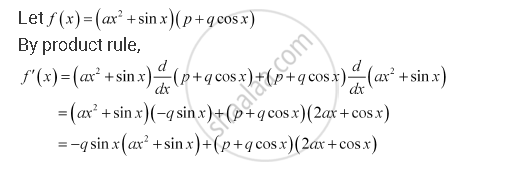Share

# Find the Derivative of the Following Functions (It is to Be Understood that A, B, C, D, P, Q, R and S Are Fixed Non-zero Constants and M and N Are Integers): (Ax2 + Sin X) (P + Q Cos X) - Mathematics

#### Question

Find the derivative of the following functions (it is to be understood that abcdp, q, r and s are fixed non-zero constants and m and n are integers): (ax2 + sin x) (p + q cos x)

#### SolutionIs there an error in this question or solution?

#### APPEARS IN

NCERT Solution for Mathematics Textbook for Class 11 (2018 (Latest))
Chapter 13: Limits and Derivatives
Q: 24 | Page no. 318

#### Video TutorialsVIEW ALL 

Solution Find the Derivative of the Following Functions (It is to Be Understood that A, B, C, D, P, Q, R and S Are Fixed Non-zero Constants and M and N Are Integers): (Ax2 + Sin X) (P + Q Cos X) Concept: Derivative - Derivative of Polynomials and Trigonometric Functions.
S